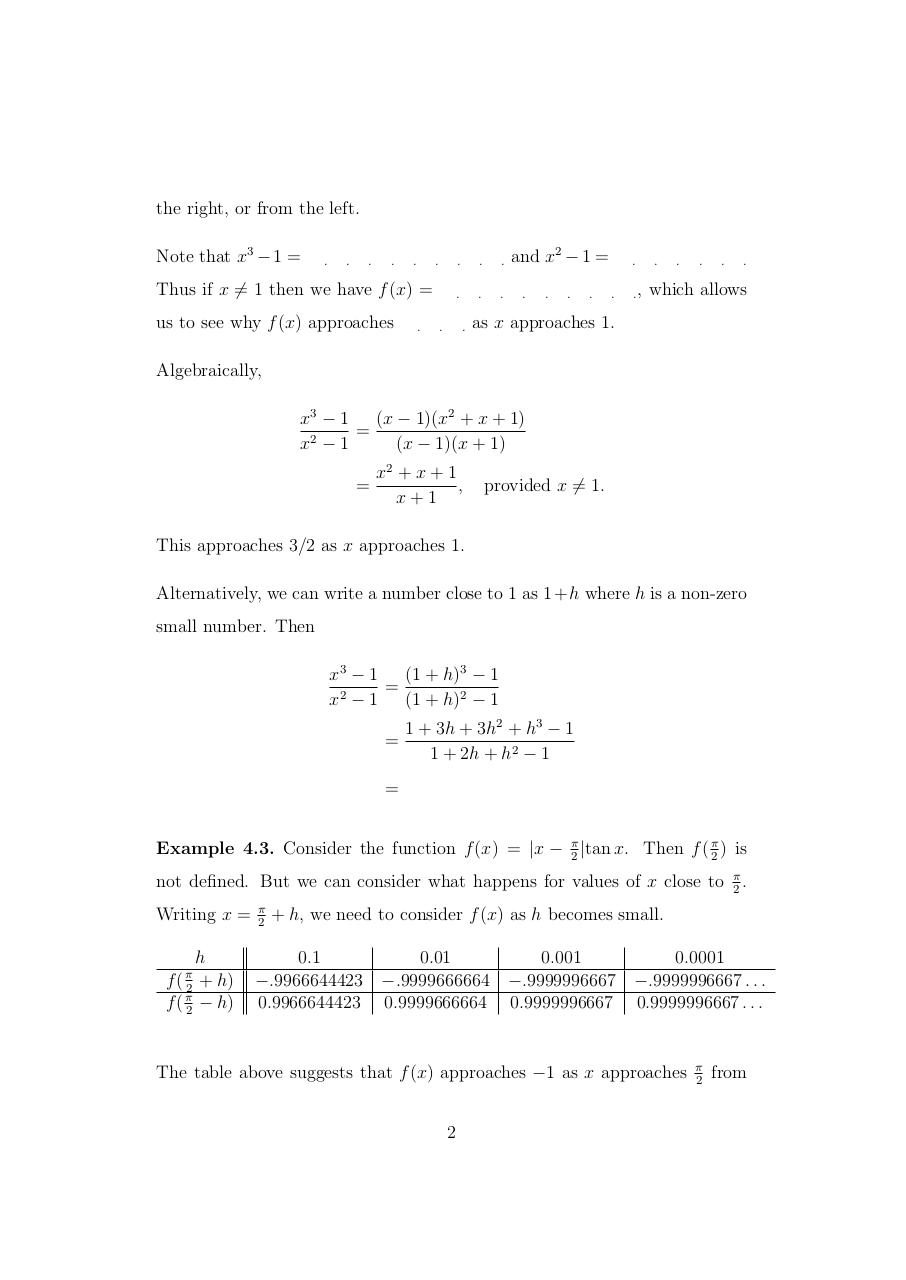# Limits.pdfPage 1 2 3 4 5 6 7 8 9 10 11 12

#### Text preview

the right, or from the left.
Note that x3 − 1 =

.

.

.

.

.

.

Thus if x 6= 1 then we have f (x) =
us to see why f (x) approaches

.

.

.

.

.

.

.

.

.

and x2 − 1 =
.

.

.

.

.
.

.,

.

.

.

.

.

which allows

as x approaches 1.

Algebraically,
x3 − 1
(x − 1)(x2 + x + 1)
=
x2 − 1
(x − 1)(x + 1)
=

x2 + x + 1
,
x+1

provided x 6= 1.

This approaches 3/2 as x approaches 1.
Alternatively, we can write a number close to 1 as 1 + h where h is a non-zero
small number. Then
x3 − 1
(1 + h)3 − 1
=
x2 − 1
(1 + h)2 − 1
=

1 + 3h + 3h2 + h3 − 1
1 + 2h + h2 − 1

=
Example 4.3. Consider the function f (x) = |x − π2 |tan x. Then f ( π2 ) is
not defined. But we can consider what happens for values of x close to
Writing x =

f ( π2
f ( π2

h
+ h)
− h)

π
2

π
.
2

+ h, we need to consider f (x) as h becomes small.

0.1
0.01
0.001
0.0001
−.9966644423 −.9999666664 −.9999996667 −.9999996667 . . .
0.9966644423 0.9999666664 0.9999996667 0.9999996667 . . .

The table above suggests that f (x) approaches −1 as x approaches
2

π
2

from